• RGB2Gray实现方案1使用MATLAB自带rgb2gray函数，实现bmp转Gray算法，这个适用于PC上的实现。1.2.1. Matlab代码clear all;% --------------------------------------------------------------------------% Rea...

1. RGB转Gray算法实现

1.1. RGB转Gray概念

1.2. RGB2Gray实现方案1

使用MATLAB自带rgb2gray函数，实现bmp转Gray算法，这个适用于PC上的实现。

1.2.1. Matlab代码

clear all;

% --------------------------------------------------------------------------

% Read an RGB image and convert it to a gray image.

h = size(IMG1,1);         % 读取图像高度

w = size(IMG1,2);         % 读取图像宽度

%-----------------------------------------------

subplot(1,2,1);    % 分为1行2列的子图显示，并且显示原图

% figure(1);

imshow(IMG1);

title('Original Image');

%-----------------------------------------------

IMG2 = rgb2gray(IMG1);

subplot(1,2,2);    % 分为1行2列的子图显示，并且显示原图

% figure(2);

imshow(IMG2);

title('Processed Image');

imwrite(IMG2,'E:\Matlab_Information\Matlab_Project\RGB2Gray_Process\PLMM_Gray.bmp');  %保存重建后的BMP

whos IMG2;  %输出转换后Gray图像信息

whos IMG3;  %输出转换完的Gray图像信息

1.2.2. 效果图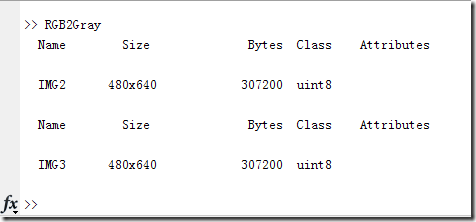可见大小变成了原来的1/3。系统直接存储为灰度格式的BMP图像。这对于后期自己实现Gray转换太有用了！1.3. RGB2Gray实现方案2

1.3.1. RGB2Gray算法分析及改进

Gray = (R^2.2 * 0.2973 + G^2.2 * 0.6274 + B^2.2 * 0.0753)^(1/2.2)

该方法运行速度稍慢，但是效果很好。

2) 原则上的灰度，就是让R=G=B，那顾名思义，可以直接求平均，如下：

GRAY = (RED+BLUE+GREEN)/3

(GRAY,GRAY,GRAY ) 替代 (RED,GREEN,BLUE)

不过这样会导致图像质量不好，这是必然的，因为也许图像的分量不均匀，再者肉眼对色彩的敏感程度也是不一样的。

3) 典型灰度转换公式

对于彩色转灰度，有一个很著名的心理学公式：

Gray = R*0.299 + G*0.587 + B*0.114

在此0.299+0.587+0.144=1，刚好是满偏，这是通过不同的敏感度以及经验总结出来的公式，一般直接用这个！

而实际应用时，希望避免低速的浮点运算，所以需要整数算法。注意到系数都是小数点后3位，我们可以将它们缩放1000倍来实现整数运算算法，如下

Gray = (R*299 + G*587 + B*114) / 1000

加上500是为了四舍五入。但是除法就是不爽，为了能在后续实现移位，将1000扩展到1024：

Gray = (R*299 + G*587 + B*114) / 1024 = Gray = (R*299 + G*587 + B*114) >>10

适当的还可以在精简，压缩到8位以内，现在变成这样子：

Gray = (R*75 + G*147 + B*36) >>8

到目前为止，整数算法已经很快了，但是完美是没有极限的，其实是可以更快的，观察原始式子

Gray = R*0.299 + G*0.587 + B*0.114

每一通道数据乘以一个常数，这三个变量可以实现算好，保存在矩阵中，这样就只是查找表的时间了，具体时间的提升，Matlab的实现上再看！

1.3.2. Matlab代码

% -----------------------------------------------------------------------

% Relized method 2：myself Algorithm realized

% Gray = (R*75 + G*147 + B*36) >>8

IMG1 = double(IMG1);    %将图片转换为双精度类型

IMG2 = zeros(h,w);      %灰度只需要二维数组就够了，因为只有一个通道

for i = 1 : h

for j = 1 : w

IMG2(i,j) = bitshift((IMG1(i,j,1)*75 + IMG1(i,j,2)*147 + IMG1(i,j,3)*36),-8);

end

end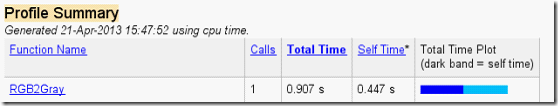1.3.3. 效果图

如下图所示，实现Gray = (R*75 + G*147 + B*36) >>8的的灰度转换，灰度大小为RGB的一半，属性啥的都OK了。1.3.4. Matlab加速运算

前面说了，这样还不够，浮点运算可以实现算好放数组里面，后期若移植FPGA直接放在ROM里面，爽半死，尤其像CPLD这种没有乘法器的，呵呵，试试看！

% -----------------------------------------------------------------------

% Gray = R*0.299 + G*0.587 + B*0.114

fp_red = fopen('RED_ARRAY.txt','w');

fp_green = fopen('GREEN_ARRAY.txt','w');

fp_blue = fopen('EBLUE_ARRAY.txt','w');

RED_ARRAY = zeros(1,256);  %存放R通道浮点运算后的数据

GREEN_ARRAY = zeros(1,256);  %存放G通道浮点运算后的数据

BLUE_ARRAY = zeros(1,256);  %存放B通道浮点运算后存放的数据

for i = 1 : 256

RED_ARRAY(1,i) = ((i - 1) * 0.299); %Matlab从1开始，但Gray从0开始

GREEN_ARRAY(1,i) = ((i - 1) * 0.587); %Matlab从1开始，但Gray从0开始

BLUE_ARRAY(1,i) = ((i - 1) * 0.114); %Matlab从1开始，但Gray从0开始

if(rem(i,16) == 0)      %取余为0，即以写入16个数据

fprintf(fp_red,'%03d ...\n',uint8(RED_ARRAY(1,i)));  %写入到TXT文件中

fprintf(fp_green,'%03d ...\n',uint8(GREEN_ARRAY(1,i)));  %写入到TXT文件中

fprintf(fp_blue,'%03d ...\n',uint8(BLUE_ARRAY(1,i)));  %写入到TXT文件中

else

fprintf(fp_red,'%03d ',uint8(RED_ARRAY(1,i)));  %写入到TXT文件中

fprintf(fp_green,'%03d ',uint8(GREEN_ARRAY(1,i)));  %写入到TXT文件中

fprintf(fp_blue,'%03d ',uint8(BLUE_ARRAY(1,i)));  %写入到TXT文件中

end

end

fclose(fp_red); %关闭文件

fclose(fp_green); %关闭文件

fclose(fp_blue); %关闭文件

以上功能可以实现三个LUT的生成，同时写入了TXT，实际运行中并不需要。此时在Matlab中填充3个数组，RED_ARRAY如下所示：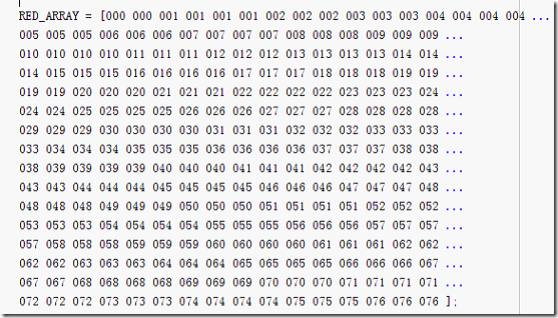如此通过查找表来实现，只要一个加法便能得到最后的结果，如下：

% -----------------------------------------------------------------------

% Relized method 3：Realized by LUT

% Gray = R*0.299 + G*0.587 + B*0.114

%      = RED_ARRAY + GREEN_ARRAY + BLUE_ARRAY

IMG1 = double(IMG1);    %将图片转换为双精度类型

IMG2 = zeros(h,w);      %灰度只需要二维数组就够了，因为只有一个通道

for i = 1 : h

for j = 1 : w

% 因为Matlab从1开始，所以+1

IMG2(i,j) = RED_ARRAY(1,IMG1(i,j,1)+1) + GREEN_ARRAY(1,IMG1(i,j,2)+1) + BLUE_ARRAY(1,IMG1(i,j,3)+1);

end

end

最终只消耗了0.196s，相对于前面的0.447s，提升了不少。关键是这样做的话，在FPGA中就完全不用浮点运算，加速逻辑的实现。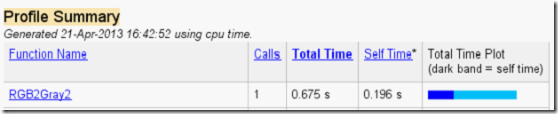展开全文• matlabrgb2gray的实现

万次阅读 2018-11-12 12:10:13
rgb2graymatlab内部一种处理图像的函数，通过消除图像色调和饱和度信息同时保留亮度实现将将RGB图像或彩色图转换为灰度图像，即灰度化处理的功能，调用这个功能的格式是I = rgb2gray(RGB)，意思是将真彩色图像RGB...

rgb2gray是matlab内部一种处理图像的函数，通过消除图像色调和饱和度信息同时保留亮度实现将将RGB图像或彩色图转换为灰度图像，即灰度化处理的功能，调用这个功能的格式是I = rgb2gray(RGB)，意思是将真彩色图像RGB转换为灰度强度图像I 。   灰度化处理有多种处理方式：分量法 最大法 平均法 加权平均法。这里，Matlab的rgb2gray函数采用的是对R、G、B分量进行加权平均的算法：

0.2989R+ 0.5870G + 0.1140B

验证程序如下（为了便于观察，实验时应选用一个RGB各分量较为明显的图像）：

clear all;clc;close all;

% 提取RGB分量

img_r = img(:,:,1);

img_g = img(:,:,2);

img_b = img(:,:,3);

figure;

subplot(2,3,1),imshow(img), title('原图');

subplot(2,3,4),imshow(img_r), title('R分量');

subplot(2,3,5),imshow(img_g), title('G分量');

subplot(2,3,6),imshow(img_b), title('B分量');

% 灰度变换,公式：f(x,y)=0.2989R+ 0.5870G + 0.1140B

img_gray1 = img(:,:,1)*0.2989+ img(:,:,2)*0.5870+ img(:,:,3)*0.1140;

subplot(2,3,2),imshow(img_gray1), title('由公式变换');

% 灰度变换,rgb2gray（）函数

img_gray2 = rgb2gray(img);

subplot(2,3,3),imshow(img_gray2), title('rgb2gray变换');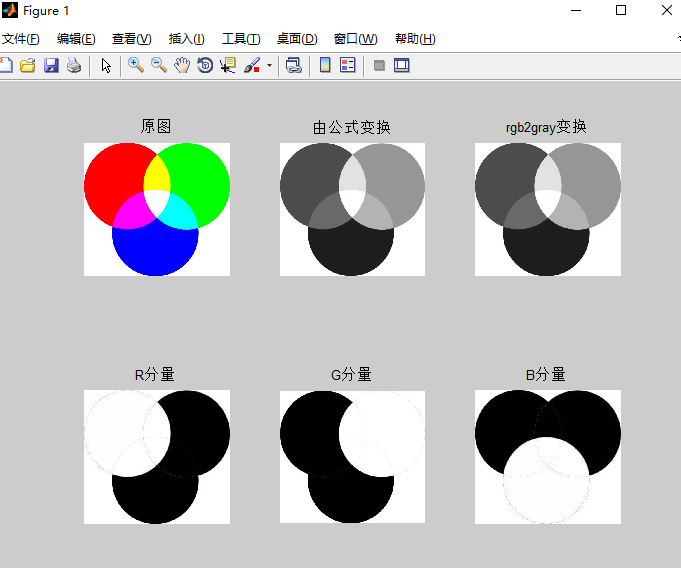上图可以看出，对于R分量图，由于G、B分量的灰度值均为0，因此G、B区域灰度值为0即为黑色区域，R分量区域灰度值均为255即为白色圆区域；同理可解释G、B分量图。

展开全文• Matlab中rgb2gray函数用法

千次阅读 2020-12-30 11:21:50
rgb2grayRGB 图像或颜色图转换为灰度图 语法 说明 示例 将 RGB 图像转换为灰度图像 将 RGB 颜色图转换为灰度颜色图 输入参数 输出参数 提示 算法 rgb2grayRGB 图像或颜色图转换为灰度图 语法 I =...

目录

语法

说明

示例

将 RGB 图像转换为灰度图像

将 RGB 颜色图转换为灰度颜色图

rgb2gray将 RGB 图像或颜色图转换为灰度图

语法

I = rgb2gray(RGB)

newmap = rgb2gray(map)

说明

I = rgb2gray(RGB) 将真彩色图像 RGB 转换为灰度图像 I。rgb2gray 函数通过消除色调和饱和度信息，同时保留亮度，来将 RGB 图像转换为灰度图。如果已安装 Parallel Computing Toolbox™，则 rgb2gray 可以在 GPU 上执行此转换。

newmap = rgb2gray(map) 返回等同于map的灰度颜色图。

示例

将 RGB 图像转换为灰度图像

读取并显示 RGB 图像，然后将其转换为灰度图。读取示例文件 peppers.png 并显示 RGB 图像。

imshow(RGB)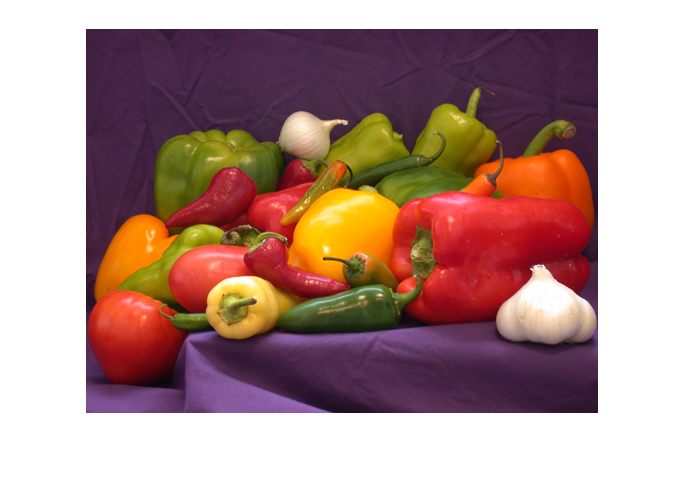将 RGB 图像转换为灰度图像并显示图像。

I = rgb2gray(RGB);
figure
imshow(I)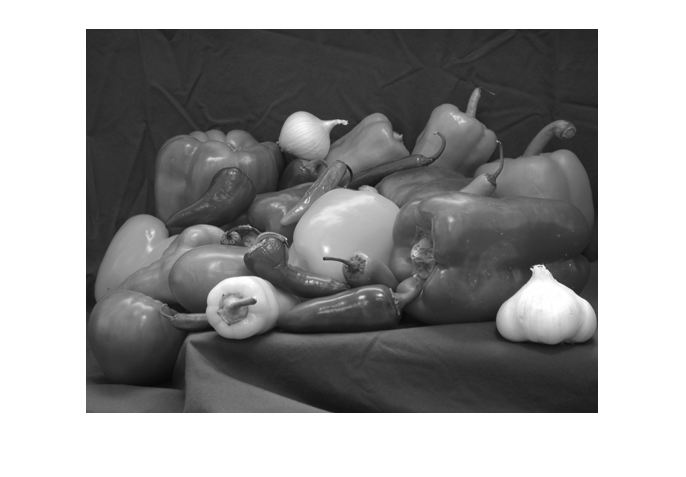将 RGB 颜色图转换为灰度颜色图

读取具有 RGB 颜色图的索引图像。然后将颜色图转换为灰度。读取示例文件 corn.tif，该文件为具有 RGB 颜色图的索引图像。

显示图像。

imshow(X,map)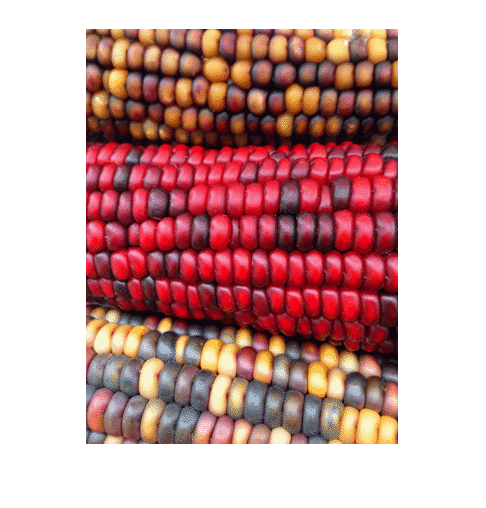将 RGB 颜色图转换为灰度颜色图并重新显示图像。

newmap = rgb2gray(map);
imshow(X,newmap)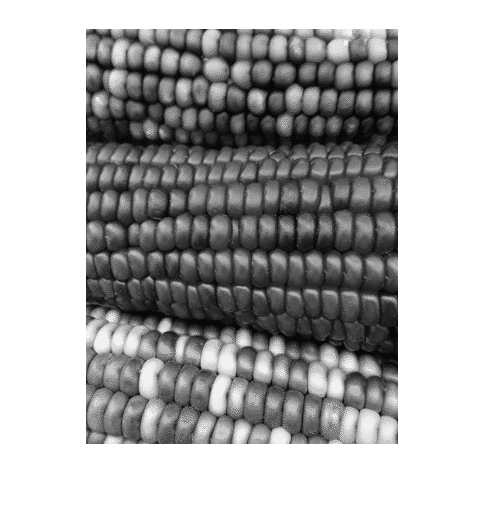RGB - 真彩色图像
m×n×3 数值数组

真彩色图像，指定为 m×n×3 数值数组。如果已安装 Parallel Computing Toolbox，则 RGB 也可以是 gpuArray。

map - 颜色图
c×3 数值矩阵

颜色图，指定为由范围 [0, 1] 内的值组成的 c×3 数值矩阵。map 的每行都是一个三元素 RGB，指定颜色图的单种颜色的红、绿和蓝分量。如果已安装 Parallel Computing Toolbox，则 map 也可以是 gpuArray。

I - 灰度图片
m×n 数值数组

灰度图像，以 m×n 数值数组形式返回。如果已安装 Parallel Computing Toolbox，则 I 也可以是 gpuArray。

newmap - 灰度颜色图
c×3 数值矩阵

灰度颜色图，返回为由范围 [0, 1] 内的值组成的 c×3 数值矩阵。newmap 的三列是相同的，因此map的每行都指定一个强度值。如果已安装 Parallel Computing Toolbox，则 newmap 也可以是 gpuArray。

提示

• rgb2gray 支持使用 MATLAB® Coder™ 生成 C 代码。

算法

rgb2gray 通过计算 R、G 和 B 分量的加权和，将 RGB 值转换为灰度值：

0.2989 * R + 0.5870 * G + 0.1140 * B

这些权重与rgb2ntsc (Image Processing Toolbox) 函数用于计算 Y 分量的权重相同。在舍入到小数点后 3 位之后，rgb2gray中用来计算灰度值的系数与Rec.ITU-R BT.601-7 中用来计算亮度 (E'y) 的系数相同。

Rec.ITU-R BT.601-7 使用以下公式计算 E'y：

0.299 * R + 0.587 * G + 0.114 * B

展开全文matlab 计算机视觉 图像处理
• matlab中基于gui rgb2grey方法 把彩图变成灰度图的方法 简单亲测好用 欢迎下载matlab 灰度
•matlab
• matlab开发-rgb2gray。不同方法转换rgb2gray的比较
• 使用Matlab给灰度图像上色的 Gray2RGB 函数，需要额外提供一张配色接近的图片。 该原始算法源于Jeny Rajan , Chandrashekar，但其运行速度过慢，我做了一些Matlab特有的小优化，大大提高了运行速度。
• HI awanjnjskmxvkxvcmxnvjmnvjmbnjnjnjn jnvjnnnkkmkmllllmatlab
• matlabrgb2gray() 的坑

千次阅读 2022-01-08 20:14:35
ima = double(rgb2gray(uint8(ima))); 特别注意在在使用rgb2gray这个函数将3通道转化成单通道的时候，必须先将这个图像转化成uint8类型。如果不进行转化的话，由于此处的ima在读取之后变成了double数据类型，直接...
ima = double(rgb2gray(uint8(ima)));

特别注意在在使用rgb2gray这个函数将3通道转化成单通道的时候，必须先将这个图像转化成uint8类型。如果不进行转化的话，由于此处的ima在读取之后变成了double数据类型，直接使用rgb2gray(ima)得到的矩阵的取值范围是在0-1范围之内的！这与造成imshow显示图像为全白的原因是一样的，imshow函数和rgb2gray函数，都会将double类型的数据视作0-1数值范围内的，如果原数据中含有大于1的数值，则这些数值都会被改为1！而imshow和rgb2gray函数把uint8类型的数据视作0-255范围之内的！

参考Imshow https://blog.csdn.net/tm807782912/article/details/46879315

展开全文matlab 计算机视觉 opencv
• matlab中 rgb2gray() 函数 具体实现

万次阅读 多人点赞 2018-06-03 20:13:46
简介 rgb2graymatlab内部一种处理图像的函数，通过消除图像色调和饱和度信息同时保留亮度实现将将RGB图像或彩色图转换为灰度图像，即灰度化处理的功能，调用这个功能的格式是I = rgb2gray(RGB)，意思是将真彩色...
• matlab开发-gray2rgb。将m x n矩阵转换为m x n x 3矩阵。
• matlabrgb2gray函数代码 使用自定义 C 代码进行边缘检测 使用 mex 函数，可以编写自定义 C 代码，然后在 MATLAB 中使用 内容 导入图片 img = imread( 'butterfly.jpg' ); imshow(img, []); 将图像转换为灰度 grayImg...
• Matlab程序，自己手动实现rgb图像灰度图像。
• RGB 图像转换为灰度图像的函数。 默认行为与 MATLAB 的（IP 工具箱）RGB2GRAY 相同，但 MRGB2GRAY 还具有其他几种转换方案。matlab
• matlab开发-mrgb2gray。函数将RGB图像转换为灰度图像。
• matlab将灰度图片彩色图片的函数，效果不错

千次阅读 热门讨论 2020-05-29 14:36:13
通过以下公式可以将RGB转换成灰度 GRAY＝0.2989*R + 0.5870*G+ 0.1140*B 在Matlab中为我们提供了rgb2gray函数，将 RGB 图像或颜色图转换为灰度图，用法为： I = rgb2gray(RGB) newmap = rgb2gray(map) I = rgb2gray...
• RGB转Gray

千次阅读 2018-03-11 16:09:43
Matlab里内置了一个函数rgb2gray()实现该功能 也可以用公式实现转化 G=0.30*R+0.59*G+0.11*B rgb_image=imread('Fig0604(a).tif'); %gray_im=zeros(600,600); %% %不用循环的做法 gray_im=rgb_image(:,:,1)*0.3...
•matlab 计算机视觉
• gray_level_conversion 使用Matlab进行RGB格式的图像灰度转换MATLAB
• Y'和Y是不同的，而Y就是所谓的流明(luminance)，表示光的浓度且为非线性，使用伽马修正(gamma correction)编码处理 rgb原图 matlab 源码： clear all; close all; clc; img = imread('lena1.jpg');%图像读入 figure,...matlab 图像处理matlab
• 这是一个简单的 m 脚本，可将 RGB 图像转换为灰度。 该代码未使用内置的 matlab 函数。 灰度转换是通过算法完成的。matlab
• 转换方法要求原图为单通道图像，所以首先使用了rgb2gray的方法。 转换原理为原图像像素值越大，在热图中就越红，反之在热图中就越蓝。 colormap(jet);使用的jet色图如下： 整个代码如下： ...RGB图像 Matlab
• MyYuanLaiPic = imread('e:/image/matlab...MyFirstGrayPic = rgb2gray(MyYuanLaiPic);%用已有的函数进行RGB到灰度图像的转换 [rows , cols , colors] = size(MyYuanLaiPic);%得到原来图像的矩阵的参数 MidGr...matlab RGB
• 出现这种情况是你使用的图像是因为是8位色深度的图片，可以使用qq截图等方式将其截图下来改为24位色深度的图片就可以解决其问题。 参考了网上的网友的说法就解决了该问题的。感谢 ......
• 我有一个RGB图像，我想转换为灰度，但我想保持一种颜色。这是我的照片：我想保持红色。其余的应该是灰度。这是我的代码输出到目前为止(你可以看到，区域是正确的，我不知道为什么他们是白色，而不是红色)：这里是我...
• MATLAB-RGB转YUV和YCbCr

千次阅读 2019-04-17 20:35:24
另外YUV格式具有亮度信息和色彩信息分离的特点，但大多数图像处理操作都是基于RGB格式，需要将RGB格式转为YUV格式。而当图像进行后期显示时，又需要将YUV转换为RGB域。 2RGB格式转为YUV格式转换公式 　 　将上述...
• 错误使用 rgb2gray>parse_inputs (line 80) MAP 必须为 m x 3 的数组。 出错 rgb2gray (line 52) isRGB = parse_inputs(X); 出错 huidujunhenghua (line 2) i=rgb2gray(i);%rgb转化为灰度图matlab...

matlabrgb转graymatlab 订阅